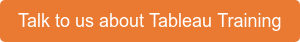# Business Intelligence Blog

## Featured Stories

Filter By Categories

One of the major subsets of analysis (or drivers for analysis) is "Is this normal behaviour?", and one of the most common chart types to address this question is the Control Chart. In short it plots a measure over time and compares this to an upper and lower "Control Limit" giving you confidence that a process or outcome is normal and/or under control.

But how do we build these in Tableau?

Option 1 - Super Fast

This option builds you a basic control chart using Mean and Standard Deviation boundaries, Rather than give you the steps I'll embed a short video showing just how quickly this can be done.

Option 2 - Simple BIY (Build it yourself)

What I mean is we are going to create the calculations and add them to our viz. This time I'm going to break down the steps and give you the code so you can simply copy and paste into your Calculation Editor window in Tableau.

The important thing to understand about Control Charts in Tableau is they use table calculations, I will endeavour to write a blog post about these but there are some great resources out there already. For this example I'm using superstore data - anyone who uses Tableau should be familiar with this data source.First we will create a simple Sales over time line chart by putting a continuous Month of Order Date on columns and Sum(Sales) on rows Next we will create the calculation we need for the average line, in simple terms we are taking the sum(Sales) for each month and averaging those values. We'll call this calculated field [Control Average] (we are going to re-use it in the next couple of calculations). `WINDOW_AVG( Sum([Sales]) )` `Upper_Control_Limit[Control Average] + WINDOW_STDEV( Sum([Sales]) )Lower_Control_Limit[Control Average] - WINDOW_STDEV( Sum([Sales]) )` Now we add in the upper and lower control limits, again using a table calculation for standard deviations. The only difference between the two being the addition or subtraction of the standard deviation from the average. The next step is to add these fields to the view, we don't add them to rows or Columns, but instead to "Detail" on the marks card. The triangle (or delta) on each field shows us it is a table calculation and therefore we need to be wary of scope and direction. For the purpose of this chart the default setting of Table Across is perfect. (Table=Scope, Direction=Across)To add these calculated values to the viz we right click on the numeric axis (in this example the rows axis) and "Add Reference Line" once in the reference line dialog box set the first one as the image here.. When adding the upper and lower control limits you can simply follow the same process as for the average line two more times, however my preference is to do this as a single reference band.If you have followed the above instructions you should have a chart that looks like this;But why go to the trouble of creating calculations when you can simply drag and drop the elements you need from the analytics pane, in short, because we can then use them for other purposes.

The second blog post in this series is now available - Add parameterised control limits and highlight outliers!

If you want more help with advanced skills in Tableau we have a number of Tableau Accredited Trainers.### Popular Stories

Process Control Charts in Tableau